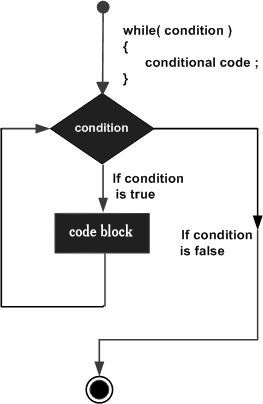Java 循环之 while 语句

语法

while 是最基本的循环，它的语法一般如下

```while( 布尔表达式 ) {

// 循环体
}
```

Java while 循环流程图范例

```public class Test {
public static void main(String args[]) {
int x = 10;
while( x < 20 ) {
System.out.print("value of x : " + x );
x++;
System.out.print("\n");
}
}
}
```

```value of x : 10
value of x : 11
value of x : 12
value of x : 13
value of x : 14
value of x : 15
value of x : 16
value of x : 17
value of x : 18
value of x : 19
```

Java 基础教程

简单教程，简单编程 - IT 入门首选站